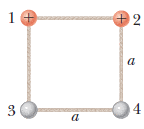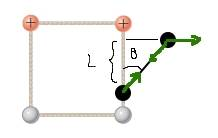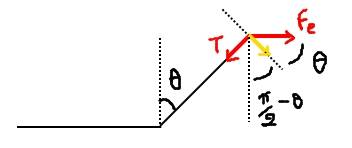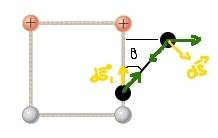# Seemingly a related rates problem

Homework Statement:
Four balls of equal mass lie on a horizontal, frictionless, nonconducting surface. They are connected by a lightweight, nonconducting strings of length ##a## as in the figure. Two have a charge ##+q##.
If we cut the string between the two charges, what would be the maximum speed of the uncharged balls?
Relevant Equations:
.The way I imagine it is that the two uncharged balls will move only vertically, while the charged balls will be coming down, and getting away from each other horizontally.
If I focus on just one side, then I notice that ##L## is decreasing from above and below by the same amount (same vertical force component).
I'll fix a reference reference frame on the uncharged balls, and so ##\theta## will be the angle between the vertical and the string between the balls.
I will directly remove second powers of differentials.\begin{align*} a^2&=(L+L'd\theta)^2+(a\sin\theta+d(a\sin\theta))^2\\ a^2&=L^2+2LL'd\theta+(a\sin\theta)^2+2a^2\sin\theta\cos\theta d\theta\\ 0&=2(a\cos\theta)L'd\theta+2a^2\sin\theta\cos\theta d\theta\\ L'&=-a\sin\theta \end{align*}And so the maximum speed is ##v=\frac a2##, since ##L'## accounts for the vertical movement of both charges.
I feel that this is wrong, though, and that I should be using an energy approach ..
Any guidance? Thank you!

haruspex
Homework Helper
Gold Member
2020 Award
I haven't tried to understand where you get those equations from, but I note that you are defining L' as ##\frac{dL}{d\theta}##. How do you get from there to a derivative wrt time?
Isn't it obvious that the magnitude of the charges would affect the speed?

I haven't tried to understand where you get those equations from
Since ##a## is constant, I used the pythagorean theorem with ##L## and the opposite side to ##\theta##.
How do you get from there to a derivative wrt time?
Isn't it obvious that the magnitude of the charges would affect the speed?
Right.. This would become ##L'(\theta(t))=-a\dot\theta(t)\sin\theta(t)##, though.
I can use ##\tau=I\ddot\theta(t)##, with ##\tau=-aF_e(t)\cos\theta(t)##, about the uncharged ball, but I don't think that that is a doable differential equation..

OK.. The decrease in potential energy of one charge is ##-\Delta U=-(\frac{kq^2}{r}-\frac{kq^2}{a})##, with ##r=a+2a\sin\theta(t)##, hence$$v_c^2=-\frac2m\left(\frac{kq^2}{a+2a\sin\theta(t)}-\frac{kq^2}{a}\right)=\frac{4kq^2}{am}\frac{\sin\theta(t)}{1+2\sin\theta(t)}$$The speed in the vertical direction can be found by multiplying by ##\sin\theta##:$$v_c\sin\theta(t)=\sqrt{\frac{4kq^2}{am}\frac{\sin^3\theta(t)}{1+2\sin\theta(t)}}$$which I need to maximize, then divide by two.
I don't care about when it is maximum, I only care about the angle..
What do you think?

haruspex
Homework Helper
Gold Member
2020 Award
I used the pythagorean theorem with L and the opposite side to θ.
Oh, all that was to get ##L'=-a\sin(\theta)##? Why not just write ##L=a\cos(\theta)## and differentiate?
become L′(θ(t))=−aθ˙(t)sin⁡θ(t), though.
I think you mean ##\dot L=L'\dot\theta=-a\sin(\theta)\dot\theta##.
The decrease in potential energy of one charge
Isn't that the decrease in PE of the system?
And that has to be shared between four balls, not all moving at the same speed.

Oh, all that was to get ##L'=-a\sin(\theta)##? Why not just write ##L=a\cos(\theta)## and differentiate?Alright, fix a reference frame to the lower right uncharged ball.The charged ball experiences a tension, and ##F_e(t)=kq^2/(a+2a\sin\theta(t))^2##. It is going to move in a circle.
The work done by the net force is\begin{align*} W&=\int(F_e\cos\theta)ad\theta\\ &=\frac{kq^2}{a}\int_0^{t'}\frac{\theta'(t)\cos\theta(t)}{\left(1+2\sin\theta(t)\right)^2}dt\\ &=\frac{kq^2}{2a}\int_0^{1+2\sin\theta(t')}\frac{du}{u^2}\\ &=\frac{kq^2}{a}\frac{\sin\theta(t')}{1+2\sin\theta(t')} \end{align*}
I find the speed using the kinetic energy term, get its vertical component by multiplying by ##\cos(\pi/2-\theta(t))=\sin\theta(t)##, then maximize it and divide by ##2##.
Is it the good approach?

haruspex
Homework Helper
Gold Member
2020 Award
fix a reference frame to the lower right uncharged ball.
It is very easy to go wrong when tying references frames to points that accelerate. It's late here, and I would not trust myself to judge the correctness of that workng.
Your previous approach was fine, you just had to get the relationship between the four velocities.

It is very easy to go wrong when tying references frames to points that accelerate. It's late here, and I would not trust myself to judge the correctness of that workng.
Your previous approach was fine, you just had to get the relationship between the four velocities.
##v## for charged balls, ##u## for the others.
Alright, since that's the PE of the system (two charged balls), then$$\frac12mv^2+\frac12mv^2=-\left(\frac{kq^2}{a+2a\sin\theta(t)}-\frac{kq^2}{a}\right)=\frac{2kq^2}{a}\frac{\sin\theta(t)}{1+2\sin\theta(t)}$$The other relation would be ##v_y=u##, correct?
I gave myself the freedom to fix the reference frame to an uncharged ball because it gives me the advantage of a circular path for the charged one, and it doesn't change the direction of the electric force because of the symmetry.

Steve4Physics
Homework Helper
Gold Member
.
The way I imagine it is that the two uncharged balls will move only vertically, while the charged balls will be coming down, and getting away from each other horizontally.
.
Any guidance? Thank you!

Hi. There’s a much simpler/quicker approach. We can use symmetry, conservation of momentum and conservation of energy.

Best not to use the terms 'vertically' and 'horizontally' because the balls are on a horizontal surface. Simplest to use +x, -x, +y and -y for directions in a horizontal plane.

Let's break it down a bit.

a) When the string is cut, balls 1 and 2 will swing outwards on curved paths as you describe. And balls 3 and 4 will move in the +y direction as you also describe (because of symmetry and conservation of momentum).

b) How will the four balls be arranged when the system has minimum potential energy? And how much potential energy has been lost changing to this arrangement?

c) Why must the speeds of all four balls be the same in this (minimum potential energy) arrangement?

d) Why must the speeds of balls 3 and 4 be maximum in this arrangement?

e) Can you now write an equation for the max. kinetic energy of ball 3 or ball 4?

•haruspex
haruspex
Homework Helper
Gold Member
2020 Award
##v## for charged balls, ##u## for the others.
Alright, since that's the PE of the system (two charged balls), then$$\frac12mv^2+\frac12mv^2=-\left(\frac{kq^2}{a+2a\sin\theta(t)}-\frac{kq^2}{a}\right)=\frac{2kq^2}{a}\frac{\sin\theta(t)}{1+2\sin\theta(t)}$$The other relation would be ##v_y=u##, correct?
I gave myself the freedom to fix the reference frame to an uncharged ball because it gives me the advantage of a circular path for the charged one, and it doesn't change the direction of the electric force because of the symmetry.
I guess one of those v's on the left should be a u.
You are still equating the whole of the lost potential energy to the KE gained by just two balls.
But @Steve4Physics has much the better approach.

b) How will the four balls be arranged when the system has minimum potential energy? And how much potential energy has been lost changing to this arrangement?
They should be in a straight line and, for the charged balls$$-\Delta U=-\left(\frac{kq^2}{3a}-\frac{kq^2}{a}\right)$$plus the work done by the tension for each couple, which do not appear to cancel since the displacements do not make opposite angles with respect to pi/2 with said force.c) Why must the speeds of all four balls be the same in this (minimum potential energy) arrangement?
Momentum is conserved, and so we have$$2\vec v+2\vec u=\vec0\Leftrightarrow v=u$$since, at the end, each couple's velocities are in the same direction.
d) Why must the speeds of balls 3 and 4 be maximum in this arrangement?
Because that of 1 and 2 are maximum
e) Can you now write an equation for the max. kinetic energy of ball 3 or ball 4?
I guess that what should be written is ##\Delta K=mv^2+mu^2=2mu^2=-\Delta U##, right? But, as I mentioned in b), I don't see how ##-\Delta U## is for the whole system..

Thank you!

But, as I mentioned in b), I don't see how ##-\Delta U## is for the whole system..
For 3 and 4, ##dW_{34}=-\vec T\cdot d\vec s-\vec{T'}\cdot d\vec s##.
For 1 and 2, ##dW_{12}=\vec{T}\cdot d\vec{s'}+\vec{T'}\cdot d\vec{s''}+\vec F_e\cdot(d\vec s'-d\vec{s''})##, so\begin{align*} dW_S&=\vec T\cdot(d\vec{s'}-d\vec s)+\vec{T'}\cdot(d\vec{s''}-d\vec s)-dU\\ &=-dU\\ \end{align*}and that's why we don't care about the tensions; their work is zero in the frames relative to 3 and 4..
At the end\begin{align*} mv^2+mu^2&=2mu^2\\ &=-\left(\frac{kq^2}{3a}-\frac{kq^2}{a}\right)\\ &=\frac{2kq^2}{3a}\\ u&=\sqrt{\frac{kq^2}{3ma}} \end{align*}
I guess that that's it.. thank you @haruspex and @Steve4Physics!

Last edited:
Steve4Physics
Homework Helper
Gold Member
Well done. My solution would have been less formal/mathematical. It might help you to see it:

The system has least potential energy when the charges are as far apart as possible (balls in a straight line: 1342). Charge separation has changed from a to 3a so potential energy lost is $\frac{kq^2}{a} - \frac{kq^2}{3a} = \frac{2kq^2}{3a}$

To maintain symmetry, and keep the system momentum zero, the balls must all have the same speed, v when in the straight line position. Balls 1 and 2 move in the -y direction and balls 3 and 4 move in the +y direction.

v is the maximum value of speed for balls 3 and 4 because they have been subject only to y-direction acceleration (from component of tension) from rest.

Since Kinetic energy gained = potential energy lost
$4(\frac{1}{2}mv^2) = \frac{2kq^2}{3a}$
which gives the value for v.

•archaic